StatLect

# Normal distribution - Maximum Likelihood Estimation

This lecture deals with maximum likelihood estimation of the parameters of the normal distribution.

Before continuing, you might want to revise the basics of maximum likelihood estimation (MLE).## Assumptions

Our sample is made up of the firstterms of an IID sequenceof normal random variables having meanand variance.

The probability density function of a generic term of the sequence isThe meanand the varianceare the two parameters that need to be estimated.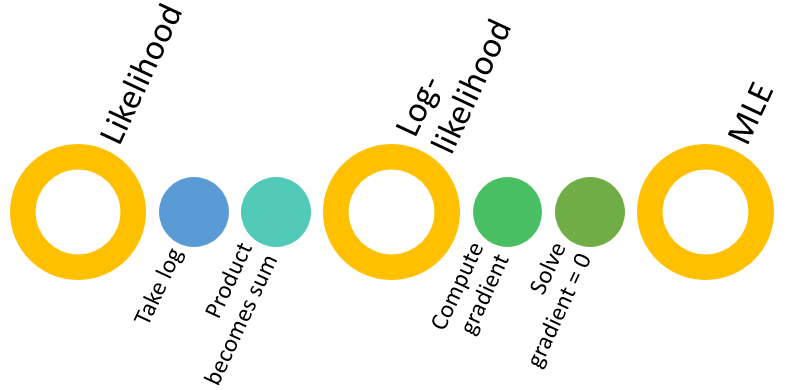## The likelihood function

The likelihood function isProof

Given the assumption that the observations from the sample are IID, the likelihood function can be written as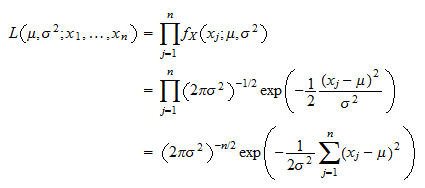## The log-likelihood function

The log-likelihood function isProof

By taking the natural logarithm of the likelihood function, we get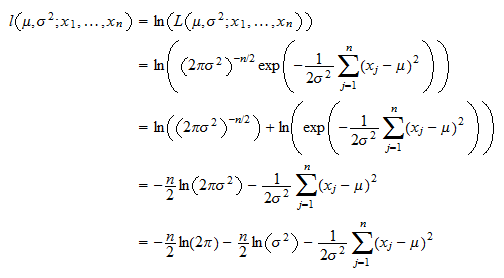## The maximum likelihood estimators

The maximum likelihood estimators of the mean and the variance areProof

We need to solve the following maximization problemThe first order conditions for a maximum areThe partial derivative of the log-likelihood with respect to the mean iswhich is equal to zero only ifTherefore, the first of the two first-order conditions impliesThe partial derivative of the log-likelihood with respect to the variance iswhich, if we rule out, is equal to zero only ifThus, the system of first order conditions is solved byThus, the estimatoris equal to the sample mean and the estimatoris equal to the unadjusted sample variance.

## Asymptotic variance

The vectoris asymptotically normal with asymptotic mean equal toand asymptotic covariance matrix equal toProof

The first entry of the score vectorisThe second entry of the score vector isIn order to compute the Hessian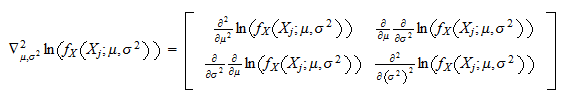we need to compute all second order partial derivatives. We haveandFinally,which, as you might want to check, is also equal to the other cross-partial derivative. Therefore, the Hessian isBy the information equality, we have that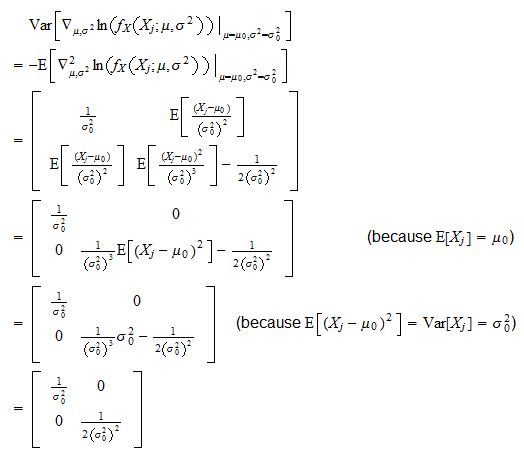As a consequence, the asymptotic covariance matrix is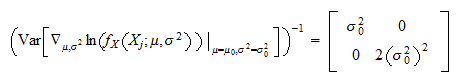In other words, the distribution of the vectorcan be approximated by a multivariate normal distribution with meanand covariance matrix## Other examples

StatLect has several pages that contain detailed derivations of MLEs. Learn how to find the estimators of the parameters of the following distributions and models.

TypeSolution
Exponential distributionUnivariate distributionAnalytical
Poisson distributionUnivariate distributionAnalytical
T distributionUnivariate distributionNumerical
Multivariate normal distributionMultivariate distributionAnalytical
Gaussian mixtureMixture of distributionsNumerical (EM)
Normal linear regression modelRegression modelAnalytical
Logistic classification modelClassification modelNumerical
Probit classification modelClassification modelNumerical